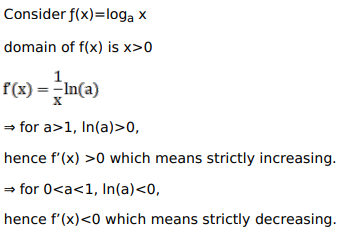# Solve this following

Question:

Prove that the function $f(\mathrm{x})=\log _{\mathrm{a}} \mathrm{x}$ is strictly increasing on $] 0, \infty[$ when $\mathrm{a}>1$ and strictly decreasing on ] $0, \infty[$ when \$0

Solution: